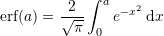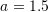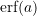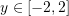### Mathematica Lab Tutorials: Lab 10

### Lab Questions

Consider the following integralwith• Use the built-in numerical integration “NIntegrate” function to calculate an approximation to the true value of the integral. Consider this to be the true value.
• Plotfor• Using the midpoint rectangle rule, provide a table with the following columns: n,h,I_2,|E| with n=1,2,4,8,16,…,128
• Using the trapezoidal rule, provide a table with the following columns: n,h,I_T,|E| with n=1,2,4,8,16,…,128
• Using Simpson’s 1/3 rule, provide a table with the following columns: n,h,I_S1,|E| with n=1,2,4,8,16,…,128
• Using Simpson’s 3/8 rule, provide a table with the following columns: n,h,I_S2,|E| with n=1,2,4,8,16,…,128
• Comment on the accuracy obtained using each method.

### Video Tutorials

Midpoint, Terapezoidal, Simpson’s 1/3, and Simpson’s 3/8 rules